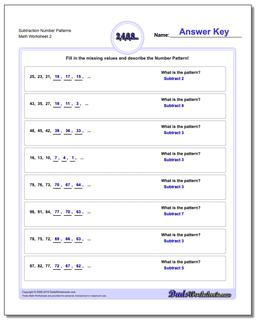# Math Worksheets: Number Patterns: Number Patterns: Subtraction Number Patterns (Second Worksheet)## Subtraction Number Patterns (Second Worksheet)

PropertyValue
DescriptionSubtraction Number Patterns: Number pattern problems using only subtraction operations. (Second Worksheet)
Resource TypeWorksheet